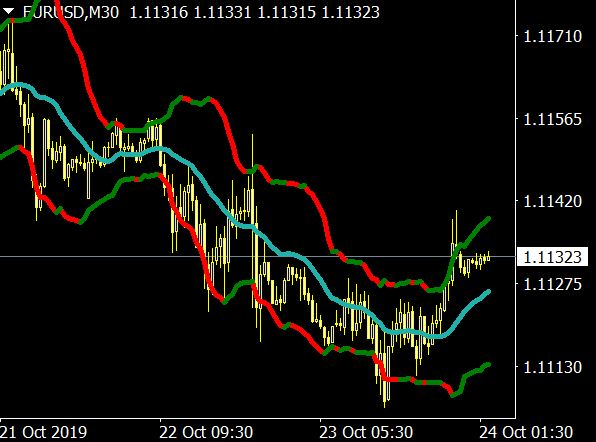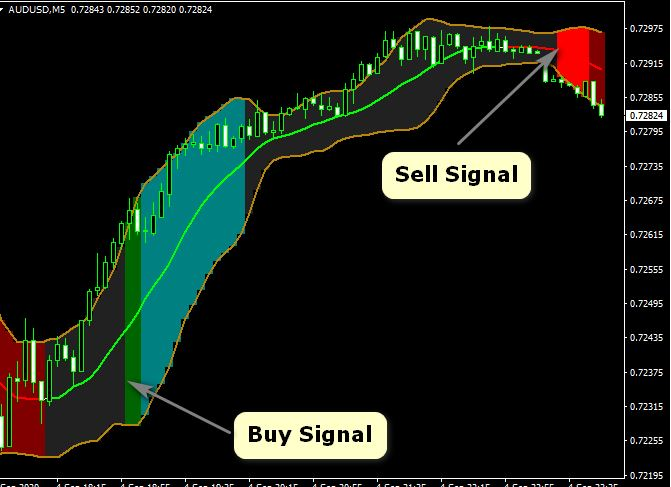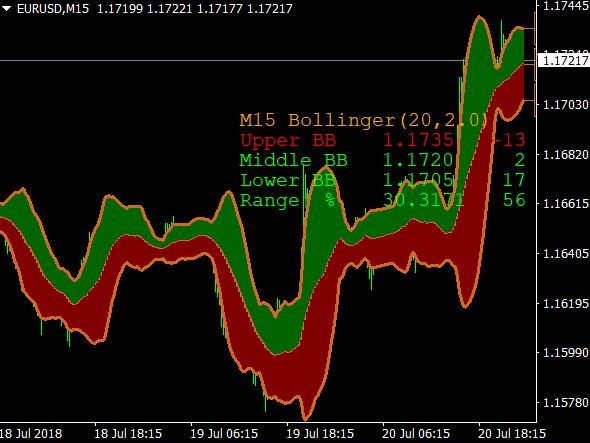Sunday , September 24 2023

The Bollinger Bands strategy is a simple, yet effective, Bollinger Bands strategy can be used to identify market trends and potential trade entry and exit points. The strategy is based on the premise that price action tends to remain within a certain range, with upper and lower limits set by the Bollinger Bands.

## How to calculate Bollinger Bands

Bollinger Bands are a best forex trading indicator that is used to measure market volatility. The bands are comprised of an upper and lower band, which are placed 2 standard deviations away from a simple moving average.First, you will need to calculate the simple moving average (SMA) of the security’s price over a certain period of time.

To do this, simply add together all of the prices over the specified time period and divide by the number of prices. For example, if the security’s price was \$10 on Monday, \$12 on Tuesday, and \$11 on Wednesday, the SMA would be (10+12+11)/3 = 11.

## Bollinger Band squeeze Breakout Strategy PDF

There are a number of different Bollinger Band trading strategies that can be employed, depending on the trader’s objectives. One common Bollinger Band strategy is to look for price action to break out above or below the upper or lower Bollinger Bands.

## Indicators to combine with Bollinger Bands

The most common Bollinger Bands strategy is to combine indicators with Bollinger Bands. There are many different indicators that can be used, but some of the most popular include:The Relative Strength Index (RSI): The RSI is a momentum indicator that measures how fast price is moving up or down. If the RSI is above 70, it means price is moving up quickly and it might be time to sell. If the RSI is below 30, it means price is moving down quickly and it might be time to buy.

The Stochastic Oscillator: The Stochastic Oscillator is another momentum indicator that measures how fast price is moving up or down. If the Stochastic Oscillator is above 80, it means price is moving up quickly and it might be time to sell. If the Stochastic Oscillator is below 20, it means price is moving down quickly and it might be time to buy.

Moving Averages: Moving averages are a trend indicator that show you whether price is trending up or down. If price is above the 200-day moving average.

### Bollinger Bands vs Keltner Channel Mt4 Indicator

The bands are created by plotting a simple moving average (SMA) in the middle of the chart and then adding and subtracting a standard deviation BBands Stop from that average.This creates an upper and lower band around the SMA. The space between the two bands is referred to as the “bandwidth”.

### Bollinger Bands vs moving average Indicator

They are created by using a moving average and adding/subtracting two standard deviations from that moving average.